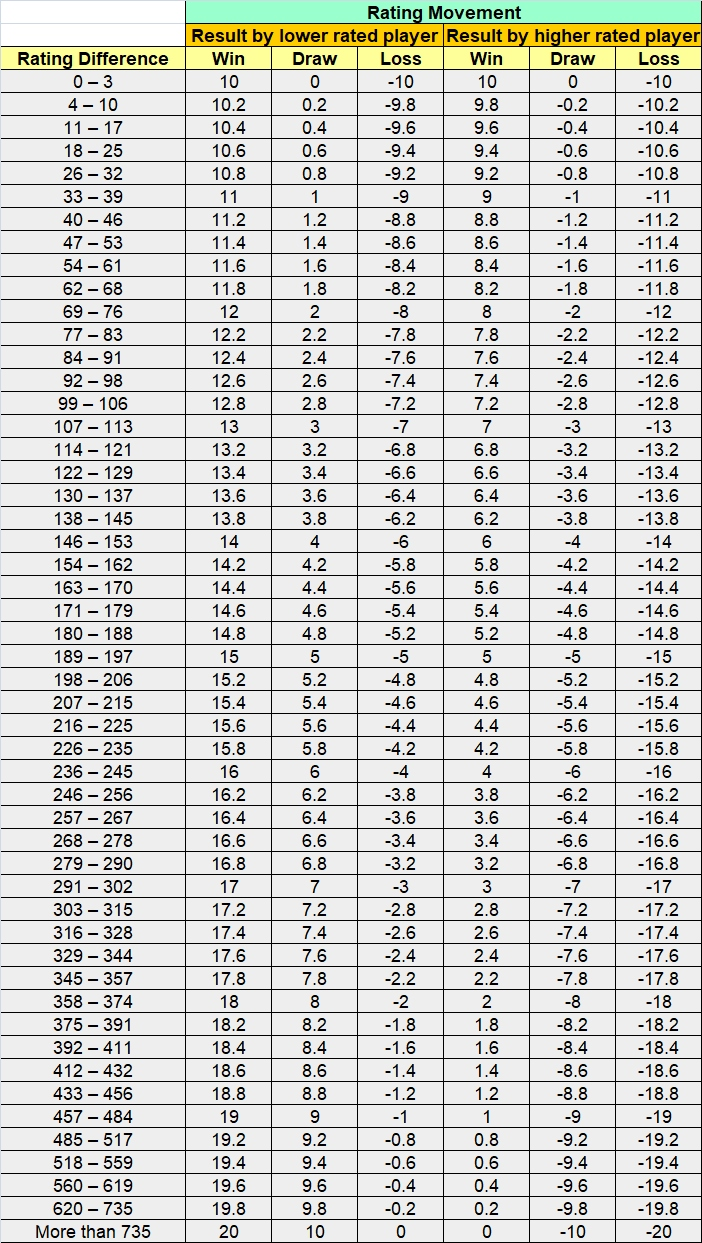## Your New Monthly ECF Rating Explained

### Introduction

The ECF grading system was the method formerly used by the English Chess Federation (ECF) to indicate a player’s strength. It was first published in 1958 and was devised by Richard W. B. Clarke, father of politician Charles Clarke. The 3 digit ECF grades were updated on a six monthly cycle, based on results towards the end of June and December. Before 2012, grades were published annually.

In July 2020 the ECF moved to 4 digit monthly ratings using a modified Elo system. These were first published in September 2020.  The final ECF grades were calculated then converted to the initial ratings as follows

Rating = 7.5 x Grade + 700

As no over the board games were being played when these ratings were first introduced due to the pandemic, the change went largely unnoticed. However, local rated matches began about a month ago and many local players are now asking how their monthly ratings are being calculated. Here is an explanation.

### The Full Explanation

Firstly, there is an ECF article that explains in detail the calculation of ratings.  Ignoring partial (provisional) ratings and keeping the calculation simple, a players new rating for the month is given by…

new rating (R1) = his/her old rating (R0) + 20 (k) x (Actual results less expected results).

The expected results are taken from table 8.1b in the FIDE Handbook referred to in the ECF article (https://handbook.fide.com/chapter/B022017).  For a junior, the k factor could be 40 instead of 20.

You can see how the calculations have affected your rating by clicking on your rating whilst in the ECF rating website.  You can see an example for Richard Ursell here (he had a good month).

### Quick Method

The calculation looks complex but can be explained more simply using the table below, which has already taken account of a k-factor of 20.

To use the table look up the rating difference between opponents on the left hand side to pick the row, and pick the correct column for the result and player. The number at the intersection is your rating change (movement) from that one game.  To get your total monthly change, simply add up all the rating changes from your games in the month.

Juniors with strong results will be given a k-factor of 40 so should double all the rating change values in the table.

There are a few illustrative examples at the end based on only one game being played in the month between two adults.### Examples

These examples use the method described in the ECF article but you will be able to see that they agree with the results from the Quick Method table.

• If there are 2 players of equal rating and one player wins then their increase using the above formula is 20 * (1 – 0.5) = 10 rating points. If the game is drawn then there is no change.
• If one player is rated 100 points more than the other player and the lower rated player wins then using the 8.1b table in the link above the increase/decrease would be 20 * (1 – 0.36) = 12.8 rating points.
• If one player is rated 100 points more than the other player and the game is drawn then the increase/decrease would be 20 * (0.5 – 0.36) = 2.8 rating  points.
• If one player is rated 100 points more than the other player and the higher rated player wins then the increase/decrease would be 20 * (1 – 0.64) = 7.2 rating points.
• If one player is rated 200 points more than the other player and the lower rated player wins then the increase/decrease would be 20 * (1 – 0.24) = 15.2 rating points.
• If one player is rated 200 points more than the other player and the game is drawn then the increase/decrease would be 20 * (0.5 – 0.24) =  5.2 rating points.
• If one player is rated 200 points more than the other player and the higher rated player wins then the increase/decrease would be 20 * (1 – 0.76) =  4.8 rating points.

The most rating points a player should gain or lose per game is 20 unless you are a junior when it could be double.

Martin Simons – B&DCL League Controller – November 2021